Seven

EVALUATION

Introduction

Much effort and research has gone into solving the problem of evaluation of information retrieval systems. However, it is probably fair to say that most people active in the field of information storage and retrieval still feel that the problem is far from solved. One may get an idea of the extent of the effort by looking at the numerous survey articles that have been published on the topic (see the regular chapter in the Annual Review on evaluation). Nevertheless, new approaches to evaluation are constantly being published (e.g. Cooper; Jardin and van Rijsbergen; Heine).

In a book of this nature it will be impossible to cover all work to date about evaluation. Instead I shall attempt to explicate the conventional, most commonly used method of evaluation, followed by a survey of the more promising attempts to improve on the older methods of evaluation.

To put the problem of evaluation in perspective let me pose three questions: (1) Why evaluate? (2) What to evaluate? (3) How to evaluate? The answers to these questions pretty well cover the whole field of evaluation. There is much controversy about each and although I do not wish to add to the controversy I shall attempt an answer to each one in turn.

The answer to the first question is mainly a social and economic one. The social part is fairly intangible, but mainly relates to the desire to put a measure on the benefits (or disadvantages) to be got from information retrieval systems. I use 'benefit' here in a much wider sense than just the benefit accruing due to acquisition of relevant documents. For example, what benefit will users obtain (or what harm will be done) by replacing the traditional sources of information by a fully automatic and interactive retrieval system? Studies to gauge this are going on but results are hard to interpret. For some kinds of retrieval systems the benefit may be more easily measured than for others (compare statute or case law retrieval with document retrieval). The economic answer amounts to a statement of how much it is going to cost you to use one of these systems, and coupled with this is the question 'is it worth it?'. Even a simple statement of cost is difficult to make. The computer costs may be easy to estimate, but the costs in terms of personal effort are much harder to ascertain. Then whether it is worth it or not depends on the individual user.

It should be apparent now that in evaluating an information retrieval system we are mainly concerned with providing data so that users can make a decision as to (1) whether they want such a system (social question) and (2) whether it will be worth it. Furthermore, these methods of evaluation are used in a comparative way to measure whether certain changes will lead to an improvement in performance. In other words, when a claim is made for say a particular search strategy, the yardstick of evaluation can be applied to determine whether the claim is a valid one.

The second question (what to evaluate?) boils down to what can we measure that will reflect the ability of the system to satisfy the user. Since this book is mainly concerned with automatic document retrieval systems I shall answer it in this context. In fact, as early as 1966, Cleverdon gave an answer to this. He listed six main measurable quantities:

(1) The coverage of the collection, that is, the extent to which the system includes relevant matter;

(2) the time lag, that is, the average interval between the time the search request is made and the time an answer is given;

(3) the form of presentation of the output;

(4) the effort involved on the part of the user in obtaining answers to his search requests;

(5) the recall of the system, that is, the proportion of relevant material actually retrieved in answer to a search request;

(6) the precision of the system, that is, the proportion of retrieved material that is actually relevant.

It is claimed that (1)-(4) are readily assessed. It is recall and precision which attempt to measure what is now known as the effectiveness of the retrieval system. In other words it is a measure of the ability of the system to retrieve relevant documents while at the same time holding back non-relevant one. It is assumed that the more effective the system the more it will satisfy the user. It is also assumed that precision and recall are sufficient for the measurement of effectiveness.

There has been much debate in the past as to whether precision and recall are in fact the appropriate quantities to use as measures of effectiveness. A popular alternative has been recall and fall-out (the proportion of non-relevant documents retrieved). However, all the alternatives still require the determination of relevance in some way. The relationship between the various measures and their dependence on relevance will be made more explicit later. Later in the chapter a theory of evaluation is presented based on precision and recall. The advantages of basing it on precision and recall are that they are:

(1) the most commonly used pair;

(2) fairly well understood quantities.

The final question (How to evaluate?) has a large technical answer. In fact, most of the remainder of this chapter may be said to be concerned with this. It is interesting to note that the technique of measuring retrieval effectiveness has been largely influenced by the particular retrieval strategy adopted and the form of its output. For example, when the output is a ranking of documents an obvious parameter such as rank position is immediately available for control. Using the rank position as cut-off, a series of precision recall values could then be calculated, one part for each cut-off value. The results could then be summarised in the form of a set of points joined by a smooth curve. The path along the curve would then have the immediate interpretation of varying effectiveness with the cut-off value. Unfortunately, the kind of question this form of evaluation does not answer is, for example, how many queries did better than average and how many did worse? Nevertheless, we shall need to spend more time explaining this approach to the measurement of effectiveness since it is the most common approach and needs to be understood.

Before proceeding to the technical details relating to the measurement of effectiveness it is as well to examine more closely the concept of relevance which underlies it.

Relevance

Relevance is a subjective notion. Different users may differ about the relevance or non-relevance of particular documents to given questions. However, the difference is not large enough to invalidate experiments which have been made with document collections for which test questions with corresponding relevance assessments are available. These questions are usually elicited from bona fide users, that is, users in a particular discipline who have an information need. The relevance assessments are made by a panel of experts in that discipline. So we now have the situation where a number of questions exist for which the 'correct' responses are known. It is a general assumption in the field of IR that should a retrieval strategy fare well under a large number of experimental conditions then it is likely to perform well in an operational situation where relevance is not known in advance.

There is a concept of relevance which can be said to be objective and which deserves mention as an interesting source of speculation. This notion of relevance has been explicated by Cooper. It is properly termed 'logical relevance'. Its usefulness in present day retrieval systems is limited. However, it can be shown to be of some importance when it is related to the development of question-answering systems, such as the one recently designed by T. Winograd at Massachusetts Institute of Technology.

Logical relevance is most easily explicated if the questions are restricted to the yes-no type. This restriction may be lifted - for details see Cooper's original paper. Relevance is defined in terms of logical consequence. To make this possible a question is represented by a set of sentences. In the case of a yes-no question it is represented by two formal statements of the form 'p' and 'not-p'. For example, if the query were 'Is hydrogen a halogen element?', the part of statements would be the formal language equivalent of 'Hydrogen is a halogen element' and 'Hydrogen is not a halogen element'. More complicated questions of the 'which' and 'whether' type can be transformed in this manner, for details the reader is referred to Belnap[5,6]. If the two statements representing the question are termed component statements then the subset of the set of stored sentences is a premiss set for a component statement if an only if the component statement is a logical consequence of that subset. (Note we are now temporarily talking about stored sentences rather than stored documents.) A minimal premiss set for a component statement is one that is as small as possible in the sense that if any of its members were deleted, the component statement would no longer be a logical consequence of the resulting set. Logical relevance is now defined as a two-place relation between stored sentences and information need representations (that is, the question represented as component statements). The final definition is as follows:

A stored sentence is logically relevant to (a representation of) an information need if and only if it is a member of some minimal premiss set of stored sentences for some component statement of that need.

Although logical relevance is initially only defined between sentences it can easily be extended to apply to stored documents. A document is relevant to an information need if and only if it contains at least one sentence which is relevant to that need.

Earlier on I stated that this notion of relevance was only of limited use at the moment. The main reason for this is that the kind of system which would be required to implement a retrieval strategy which would retrieve only the logically relevant documents has not been built yet. However, the components of such a system do exist to a certain extent. Firstly, theorem provers, which can prove theorems within formal languages such as the first-order predicate calculus, have reached quite a level of sophistication now (see, for example, Chang and Lee). Secondly, Winograd's system is capable of answering questions about its simple universe blocks in natural language. In principle this system could be extended to construct a universe of documents, that is, the content of a document is analysed and incorporated into the universe of currently 'understood' documents. It may be that the scale of a system of this kind will be too large for present day computers; only the future will tell.

Saracevic has given a thorough review of the notion of relevance in information science. Robertson has summarised some of the more recent work on probabilistic interpretations of relevance.

Precision and recall, and others

We now leave the speculations about relevance and return to the promised detailed discussion of the measurement of effectiveness. Relevance will once again be assumed to have its broader meaning of 'aboutness' and 'appropriateness', that is, a document is ultimately determined to be relevant or not by the user. Effectiveness is purely a measure of the ability of the system to satisfy the user in terms of the relevance of documents retrieved. Initially, I shall concentrate on measuring effectiveness by precision and recall; a similar analysis could be given for any pair of equivalent measures.

It is helpful at this point to introduce the famous 'contingency' table which is not really a contingency table at all.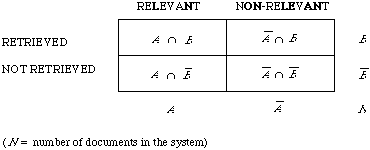A large number of measures of effectiveness can be derived from this table. To list but a few:( | . | is the counting measure)

There is a functional relationship between all three involving a parameter called generality (G) which is a measure of the density of relevant documents in the collection. The relationship is: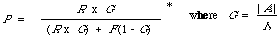For each request submitted to a retrieval system one of these tables can be constructed. Based on each one of these tables a precision-recall value can be calculated. If the output of the retrieval strategy depends on a parameter, such as rank position or co-ordination level (the number of terms a query has in common with a document), it can be varied to give a different table for each value of the parameter and hence a different precision-recall value. If [[lambda]] is the parameter, then P[[lambda]] denotes precision, R[[lambda]] recall, and a precision-recall value will be denoted by the ordered pair (R[[lambda]] , P[[lambda]] ). The set of ordered pairs makes up the precision-recall graph. Geometrically when the points have been joined up in some way they make up the precision-recall curve. The performance of each request is usually given by a precision-recall curve (see Figure 7.1). To measure the overall performance of a system, the set of surves, one for each request, is combined in some way to produce an average curve.

* For a derivation of this relation from Bayes' Theorem, the reader should consult the author's recent paper on retrieval effectiveness.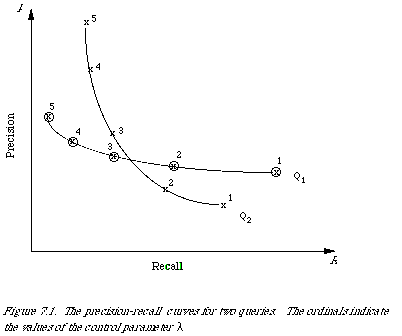Averaging techniques

The method of pooling or averaging of the individual P-R curves seems to have depended largely on the retrieval strategy employed. When retrieval is done by co-ordination level, micro-evaluation is adopted. If S is the set of requests then:where As is the set of documents relevant to request s. If [[lambda]] is the co-ordination level, then:where B[[lambda]]s is the set of documents retrieved at or above the co-ordination level [[lambda]]. The points (R[[lambda]] , P[[lambda]] ) are now calculated as follows: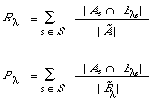Figure 7.2 shows graphically what happens when two individual P-R curves are combined in this way. The raw data are given in Table 7.1.

An alternative approach to averaging is macro-evaluation which can be independent of any parameter such as co-ordination level. The average curve is obtained by specifying a set of standard recall values for which average precision values are calculated by averaging over all queries the individual precision values corresponding to the standard recall values. Often no unique precision value corresponds exactly so it becomes necessary to interpolate.Table 7.1. THE RAW DATA FOR THE MICRO-EVALUATION IN FIGURE 7.2Interpolation

Many interpolation techniques have been suggested in the literature. See, for example, Keen.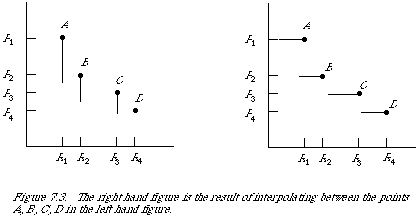Figure 7.3 shows a typical P-R graph for a single query. The points A, B, C and D, I shall call the observed points, since these are the only points observed directly during an experiment the others may be inferred from these. Thus given that A = (R1, P1) has been observed, then the next point B is the one corresponding to an increase in recall, which follows from a unit increase in the number of relevant documents retrieved. Between any two observed points the recall remains constant, since no more relevant documents are retrieved.

It is an experimental fact that average precision-recall graphs are monotonically decreasing. Consistent with this, a linear interpolation estimates the best possible performance between any two adjacent observed points. To avoid inflating the experimental results it is probably better to perform a more conservative interpolation as follows:

Let (R[[lambda]] , P[[lambda]] ) be the set of precision-recall values obtained by varying some parameter [[lambda]]. To obtain the set of observed points we specify a subset of the parameters [[lambda]]. Thus (R[[theta]] , P[[theta]] ) is an observed point if [[theta]] corresponds to a value of [[lambda]] at which an increase in recall is produced. We now have:

Gs = (R[[theta]]s, P[[theta]]s )

the set of observed points for a request. To interpolate between any two points we define:

Ps(R) = {sup P : R' >= R s.t. (R', P) [[propersubset]] Gs}

where R is a standard recall value. From this we obtain the average precision value at the standard recall value R by: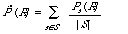The set of observed points is such that the interpolated function is monotonically decreasing. Figure 7.3 shows the effect of the interpolation procedure, essentially it turns the P-R curve into a step-function with the jumps at the observed points. A necessary consequence of its monotonicity is that the average P-R curve will also be monotonically decreasing. It is possible to define the set of observed points in such a way that the interpolate function is not monotonically decreasing. In practice, even for this case, we have that the average precision-recall curve is monotonically decreasing.

In Figure 7.4 we illustrate the interpolation and averaging process.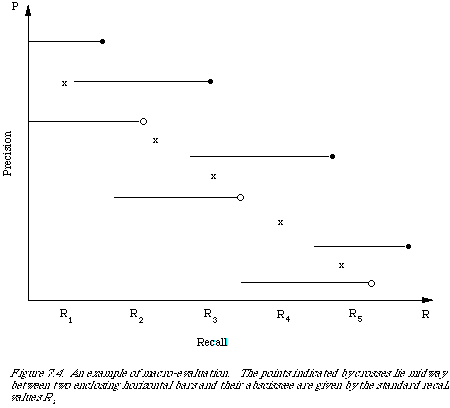Composite measures

Dissatisfaction in the past with methods of measuring effectiveness by a pair of numbers (e.g. precision and recall) which may co-vary in a loosely specified way has led to attempts to invest composite measures. These are still based on the 'contingency' table but combine parts of it into a single number measure. Unfortunately many of these measures are rather ad hoc and cannot be justified in any rational way. The simplest example of this kind of measure is the sum of precision and recall

S = P + R

This is simply related to a measure suggested by Borko

BK = P + R - 1

More complicated ones are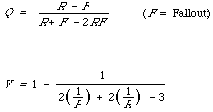Vickery's measure V can be shown to be a special case of a general measure which will be derived below.

Some single-number measures have derivations which can be justified in a rational manner. Some of them will be given individual attention later on. Suffice it here to point out that it is the model underlying the derivation of these measures that is important.

The Swets model*

As early as 1963 Swets expressed dissatisfaction with existing methods of measuring retrieval effectiveness. His background in signal detection led him to formulate an evaluation model based on statistical decision theory. In 1967 he evaluated some fifty different retrieval methods from the point of view of his model. The results of his evaluation were encouraging but not conclusive. Subsequently, Brookes suggested some reasonable modifications to Swets' measure of effectiveness, and Robertson showed that the suggested modifications were in fact simply related to an alternative measure already suggested by Swets. * Bookstein has recently re-examined this model showing how Swets implicitly relied on an 'equal variance' assumption.

It is interesting that although the Swets model is theoretically attractive and links IR measurements to a ready made and well-developed statistical theory, it has not found general acceptance amongst workers in the field.

Before proceeding to an explanation of the Swets model, it is as well to quote in full the conditions that the desired measure of effectiveness is designed to meet. At the beginning of his 1967 report Swets states:

'A desirable measure of retrieval performance would have the following properties: First, it would express solely the ability of a retrieval system to distinguish between wanted and unwanted items - that is, it would be a measure of "effectiveness" only, leaving for separate consideration factors related to cost or "efficiency". Second, the desired measure would not be confounded by the relative willingness of the system to emit items - it would express discrimination power independent of any "acceptance criterion" employed, whether the criterion is characteristic of the system or adjusted by the user. Third, the measure would be a single number - in preference, for example, to a pair of numbers which may co-vary in a loosely specified way, or a curve representing a table of several pairs of numbers - so that it could be transmitted simply and immediately apprehended. Fourth, and finally, the measure would allow complete ordering of different performances, and assess the performance of any one system in absolute terms - that is, the metric would be a scale with a unit, a true zero, and a maximum value. Given a measure with these properties, we could be confident of having a pure and valid index of how well a retrieval system (or method) were performing the function it was primarily designed to accomplish, and we could reasonably ask questions of the form "Shall we pay X dollars for Y units of effectiveness?".'

He then goes on to claim that 'The measure I proposed [in 1963], one drawn from statistical decision theory, has the potential [my italics] to satisfy all four desiderata'. So, what is this measure?

To arrive at the measure, we must first discuss the underlying model. Swets defines the basic variables Precision, Recall, and Fallout in probabilistic terms.

Recall = an estimate of the conditional probability that an item will be

retrieved given that it is relevant [we denote this P(B/A)].

Precision = an estimate of the conditional probability that an item will be

relevant given that it is retrieved [i.e. P(A/B)].

Fallout = an estimate of the conditional probability that an item will be

retrieved given that it is non-relevant [i.e. P(B/`A].

He accepts the validity of measuring the effectiveness of retrieval by a curve either precision-recall or recall-fallout generated by the variation of some control variable [[lambda]] (e.g. co-ordination level). He seeks to characterise each curve by a single number. He rejects precision-recall in favour of recall-fallout since he is unable to do it for the former but achieves limited success with the latter.

In the simplest case we assume that the variable [[lambda]] is distributed normally on the set of relevant and non-relevant documents. The two distributions are given respectively by N(u1, [[sigma]]1) and N(u2, [[sigma]]2). The density functions are given by [[florin]]1 ([[lambda]]|A) and [[florin]]2 ([[lambda]]|`A). We may picture the distribution as shown in Figure 7.5.The usual set-up in IR is now to define a decision rule in terms of [[lambda]], to determine which documents are retrieved (the acceptance criterion). In other words we specify [[lambda]]c such that a document for which the associated [[lambda]] exceeds [[lambda]]c is retrieved. We now measure the effectiveness of a retrieval strategy by measuring some appropriate variables (such as R and P, or R and F) at various values of [[lambda]]c. It turns out that the differently shaded areas under the curves in Figure 7.5 correspond to recall and fallout. Moreover, we find the operating characteristic (OC) traced out by the point (F[[lambda]], R[[lambda]]) due to variation in [[lambda]]c is a smooth curve fully determined by two points, in the general case of unequal variance, and by one point in the special case of equal variance. To see this one only needs to plot the (F[[lambda]], R[[lambda]]) points on double probability paper (scaled linearly for the normal deviate) to find that the points lie on a straight line. A slope of 45deg. corresponds to equal variance, and otherwise the slope is given by the ratio of [[sigma]]1 and [[sigma]]2. Figure 7.6 shows the two cases. Swets now suggests, regardless ofslope, that the distance 0I (actually [[radical]]20I) be used as a measure of effectiveness. This amounts to using: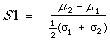which is simply the difference between the means of the distribution normalised by the average standard deviation. Unfortunately this measure does rather hide the fact that a high S1 value may be due to a steep slope. The slope, and S1, would have to be given which fails to meet Swets' second condition. We, also, still have the problem of deciding between two strategies whose OC's intersect and hence have different S1 values and slopes.

Brookes in an attempt to correct for the S1 bias towards systems with slopes much greater than unity suggested a modification to S1. Mathematically Brookes's measure is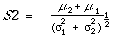Brookes also gives statistical reasons for preferring S2 to S1 which need not concern us here. Geometrically S2 is the perpendicular distance from 0 to OC (see Figure 7.6).

Interestingly enough, Robertson showed that S2 is simply related to the area under the Recall-Fallout curve. In fact, the area is a strictly increasing function of S2. It also has the appealing interpretation that it is equal to the percentage of correct choices a strategy will make when attempting to select from a pair of items, one drawn at random from the non-relevant set and one drawn from the relevant set. It does seem therefore that S2 goes a long way to meeting the requirements laid down by Swets. However, the appropriateness of the model is questionable on a number of grounds. Firstly, the linearity of the OC curve does not necessarily imply that [[lambda]] is normally distributed in both populations, although they will be 'similarly' distributed. Secondly, [[lambda]] is assumed to be continuous which certainly is not the case for the data checked out both by Swets and Brookes, in which the co-ordination level used assumed only integer values. Thirdly, there is no evidence to suggest that in the case of more sophisticated matching functions, as used by the SMART system, that the distributions will be similarly distributed let alone normally. Finally the choice of fallout rather than precision as second variable is hard to justify. The reason is that the proportion of non-relevant retrieved for large systems is going to behave much like the ratio of 'non-relevant' retrieved to 'total documents in system'. For comparative purposes 'total document' may be ignored leaving us with 'non-relevant retrieved' which is complementary to 'relevant retrieved'. But now we may as well use precision instead of fallout.

The Robertson model - the logistic transformation

Robertson in collaboration with Teather has developed a model for estimating the probabilities corresponding to recall and fallout. The estimation procedure is unusual in that in making an estimate of these probabilities for a single query it takes account of two things: one, the amount of data used to arrive at the estimates, and two, the averages of the estimates over all queries. The effect of this is to 'pull' an estimate closer to the overall mean if it seems to be an outlyer whilst at the same time counterbalancing the 'pull' in proportion to the amount of data used to make the estimate in the first place. There is now some evidence to show that this pulling-in-to-the-mean is statistically a reasonable thing to do.

Using the logit transformation for probabilities, that is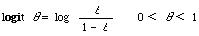the basic quantitative model for a single query j they propose is

logit [[theta]]j1 = [[alpha]]j + [[Delta]]j

logit [[theta]]j2 = [[alpha]]j - [[Delta]]j

Here [[theta]]j1 and [[theta]]j2 are probabilities corresponding to recall and fallout respectively as defined in the previous section. The parameters [[alpha]]j and [[Delta]]j are to be interpreted as follows:

[[alpha]]j measures the specificity of the query formulation; [[Delta]]j measures the separation

of relevant and non-relevant documents.

For a given query j if the query i has been formulated in a more specific way than j, one would expect the recall and fallout to decrease, i.e.

[[theta]]i1 < [[theta]]j1 and [[theta]]i2 < [[theta]]j2

Also, if for query i the system is better at separating the non-relevant from the relevant documents than it is for query j one would expect the recall to increase and the fallout to decrease, i.e.

[[theta]]i1 > [[theta]]j1 and [[theta]]i2 < [[theta]]j2

Given that logit is a monotonic transformation, these interpretations are consistent with the simple quantitative model defined above.

To arrive at an estimation procedure for [[alpha]]j and [[Delta]]j is a difficult technical problem and the interested reader should consult Robertson's thesis. It requires certain assumptions to be made about [[alpha]]j and [[Delta]]j , the most important of which is that the {[[alpha]]j }and {[[Delta]]j }are independent and normally distributed. These assumptions are rather difficult to validate. The only evidence produced so far derives the distribution of {[[alpha]]j } for certain test data. Unfortunately, these estimates, although they are unimodally and symmetrically distributed themselves, can only be arrived at by using the normality assumption. In the case of [[Delta]]j it has been found that it is approximately constant across queries so that a common-[[Delta]] model is not unreasonable:

logit [[theta]]j1 = [[alpha]]j1 + [[Delta]]

logit [[theta]]j2 = [[alpha]]j2 - [[Delta]]

From them it would appear that [[Delta]] could be a candidate for a single number measure of effectiveness. However, Robertson has gone to some pains to warn against this. His main argument is that these parameters are related to the behavioural characteristics of an IR system so that if we were to adopt [[Delta]] as a measure of effectiveness we could be throwing away vital information needed to make an extrapolation to the performance of other systems.

The Cooper model - expected search length

In 1968, Cooper stated: 'The primary function of a retrieval system is conceived to be that of saving its users to as great an extent as is possible, the labour of perusing and discarding irrelevant documents, in their search for relevant ones'. It is this 'saving' which is measured and is claimed to be the single index of merit for retrieval systems. In general the index is applicable to retrieval systems with ordered (or ranked) output. It roughly measures the search effort which one would expect to save by using the retrieval system as opposed to searching the collection at random. An attempt is made to take into account the varying difficulty of finding relevant documents for different queries. The index is calculated for a query of a precisely specified type. It is assumed that users are able to quantify their information need according to one of the following types:

(a) only one relevant document is wanted;

(b) some arbitrary number n is wanted;

(c) all relevant documents are wanted;

(4) a given proportion of the relevant documents is wanted, etc.

Thus, the index is a measure of performance for a query of given type. Here we shall restrict ourselves to Type 2 queries. For further details the reader is referred to Cooper.

The output of a search strategy is assumed to be a weak ordering of documents. I have defined this concept on page 118 in a different context. We start by first considering a special case, namely a simple ordering, which is a weak ordering such that for any two distinct elements e1 and e2 it is never the case that e1 R e2 and e2 R e1 (where R is the order relation). This simply means that all the documents in the output are ordered linearly with no two or more documents at the same level of the ordering. The search length is now defined as the number of non-relevant documents a user must scan before his information need (in terms of the type quantification above) is satisfied. For example, consider a ranking of 20 documents in which the relevant ones are distributed as in Figure 7.7. A Type 2 query with n = 2 would have search length 2, with n = 6 it would have search length 3.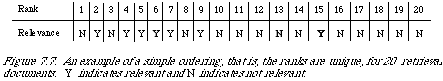Unfortunately the ranking generated by a matching function is rarely a simple ordering, but more commonly a weak ordering. This means that at any given level in the ranking, there is at l;east one document (probably more) which makes the search length inappropriate since the order of documents within a level is random. If the information need is met at a certain level in the ordering then depending on the arrangement of the relevant documents within that level we shall get different search lengths. Nevertheless we can use an analogous quantity which is the expected search length. For this we need to calculate the probability of each possible search length by juggling (mentally) the relevant and non-relevant documents in the level at which the user need is met.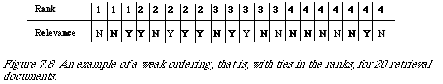For example, consider the weak ordering in Figure 7.8. If the query is of Type 2 with n = 6 then the need is met at level 3. The possible search lengths are 3, 4, 5 or 6

depending on how many non-relevant documents precede the sixth relevant document. We can ignore the possible arrangements within levels 1 and 2; their contributions are always the same. To compute the expected search length we need the probability of each possible search length. We get at this by considering first the number of different ways in which two relevant documents could be distributed among five, it is (2) = 10. Of these 4 would result in a search length of 3, 3 in a search length of 4, 2 in a search length of 5 and 1 in a search length of 6. Their corresponding probabilities are therefore, 4/10, 3/10, 2/10 and 1/10. The expected search length is now:

(4/10) . 3 + (3/10) . 4 + (2/10) . 5 + (1/10) . 6 = 4

The above procedure leads immediately to a convenient 'intuitive' derivation of a formula for the expected search length. It seems plausible that the average results of many random searches through the final level (level at which need is met) will be the same as for a single search with the relevant documents spaced 'evenly' throughout that level. First we enumerate the variables:

(a) q is the query of given type;

(b) j is the total number of documents non-relevant to q in all levels preceding the final;

(c) r is the number of relevant documents in the final level;

(d) i is the number of non-relevant documents in the final level;

(e) s is the number of relevant documents required from the final level to satisfy the need according its type.

Now, to distribute the r relevant documents evenly among the non-relevant documents, we partition the non-relevant documents into r + 1 subsets each containing i /(r + 1) documents. The expected search length is now: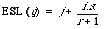As a measure of effectiveness ESL is sufficient if the document collection and test queries are fixed. In that case the overall measure is the mean expected search length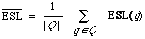where Q is the set of queries. This statistic is chosen in preference to any other for the property that it is minimised when the total expected search length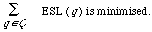To extend the applicability of the measure to deal with varying test queries and document collections, we need to normalise the ESL in some way to counter the bias introduced because:

(1) queries are satisfied by different numbers of documents according to the type of the query and therefore can be expected to have widely differing search lengths;

(2) the density of relevant documents for a query in one document collection may be significantly different from the density in another.

The first item suggests that the ESL per desired relevant document is really what is wanted as an index of merit. The second suggests normalising the ESL by a factor proportional to the expected number of non-relevant documents collected for each relevant one. Luckily it turns out that the correction for variation in test queries and for variation in document collection can be made by comparing the ESL with the expected random search length (ERSL). This latter quantity can be arrived at by calculating the expected search length when the entire document collection is retrieved at one level. The final measure is therefore:which has been called the expected search length reduction factor by Cooper. Roughly it measures improvement over random retrieval. The explicit form for ERSL is given by:where

(1) R is the total number of documents in the collection relevant to q;

(2) I is the total number of documents in the collection non-relevant to q;

(3) S is the total desired number of documents relevant to q.

The explicit form for ESL was given before. Finally, the overall measure for a set of queries Q is defined, consistent with the mean ESL, to be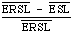which is known as the mean expected search length reduction factor.

Within the framework as stated at the head of this section this final measure meets the bill admirably. However, its acceptability as a measure of effectiveness is still debatable (see, for example, Senko). It totally ignores the recall aspect of retrieval, unless queries are evaluated which express the need for a certain proportion of the relevant documents in the system. It therefore seems to be a good substitute for precision, one which takes into account order of retrieval and user need.

For a further defence of its subjective nature see Cooper. A spirited attack on Cooper's position can be found in Soergel.

The SMART measures

In 1966, Rocchio gave a derivation of two overall indices of merit based on recall and precision. They were proposed for the evaluation of retrieval systems which ranked documents, and were designed to be independent of cut-off.

The first of these indices is normalised recall. It roughly measures the effectiveness of the ranking in relation to the best possible and worst possible ranking. The situation is illustrated in Figure 7.9 for 25 documents where we plot on the y-axis and the ranks on the x-axis.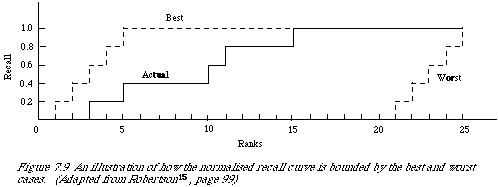Normalised recall (Rnorm) is the area between the actual case and the worst as a proportion of the area between the best and the worst. If n is the number of relevant documents, and ri the rank at which the ith document is retrieved, then the area between the best and actual case can be shown to be (after a bit of algebra):(see Salton, page 285).

A convenient explicit form of normalised recall is: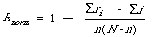where N is the number of documents in the system and N - n the area between the best and the worst case (to see this substitute ri = N - i + 1 in the formula for Ab - Aa). The form ensures that Rnorm lies between 0 (for the worst case) and 1 (for the best case).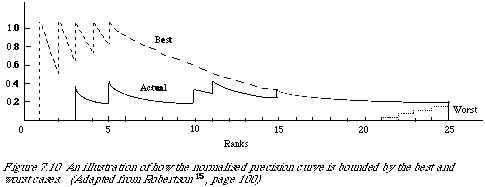In an analogous manner normalised precision is worked out. In Figure 7.10 we once more have three curves showing (1) the best case, (2) the actual case, and (3) the worst case in terms of the precision values at different rank positions.

The calculation of the areas is a bit more messy but simple to do (see Salton, page 298). The area between the actual and best case is now given by: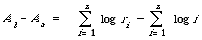The log function appears as a result of approximating [[Sigma]] 1/r by its continuous analogue [[integral]] 1/r dr, which is logr + constant.

The area between the worst and best case is obtained in the same way as before using the same substitution, and is: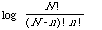The explicit form, with appropriate normalisation, for normalised precision is therefore:Once again it varies between 0 (worst) and 1 (best).

A few comments about these measures are now in order. Firstly their behaviour is consistent in the sense that if one of them is 0 (or 1) then the other is 0 (or 1). In other words they both agree on the best and worst performance. Secondly, they differ in the weights assigned to arbitrary positions of the precision-recall curve, and these weights may differ considerably from those which the user feels are pertinent (Senko). Or, as Salton (page 289) puts it: 'the normalised precision measure assigns a much larger weight to the initial (low) document ranks than to the later ones, whereas the normalised recall measure assigns a uniform weight to all relevant documents'. Unfortunately, the weighting is arbitrary and given. Thirdly, it can be shown that normalised recall and precision have interpretations as approximations to the average recall and precision values for all possible cut-off levels. That is, if R (i) is the recall at rank position i, and P (i) the corresponding precision value, then:Fourthly, whereas Cooper has gone to some trouble to take account of the random element introduced by ties in the matching function, it is largely ignored in the derivation of Pnorm and Rnorm.

One further comment of interest is that Robertson15 has shown that normalised recall has an interpretation as the area under the Recall-Fallout curve used by Swets.

Finally mention should be made of two similar but simpler measures used by the SMART system. They are:and do not take into account the collection size N, n is here the number of relevant documents for the particular test query.

A normalised symmetric difference

Let us now return to basics and consider how it is that users could simply measure retrieval effectiveness. We are considering the common situation where a set of documents is retrieved in response to a query, the possible ordering of this set is ignored. Ideally the set should consist only of documents relevant to the request, that is giving 100 per cent precision and 100 per cent recall (and by implication 0 per cent fallout). In practice, however, this is rarely the case, and the retrieved set consists of both relevant and non-relevant documents. The situation may therefore be pictured as shown in Figure 7.11, where A is the set of relevant documents, B the set of retrieved documents, and A [[intersection]] B the set of retrieved documents which are relevant.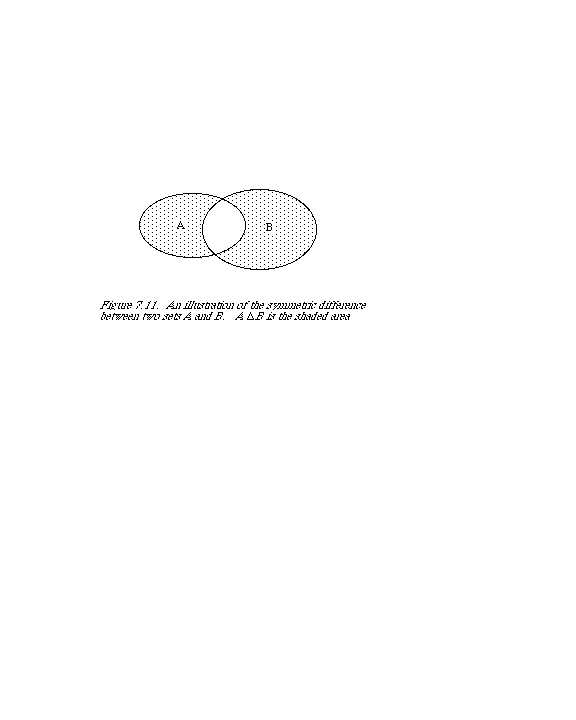Now, an intuitive way of measuring the adequacy of the retrieved set is to measure the size of the shaded area. Or to put it differently, to measure to what extent the two sets do not match. The area is in fact the symmetric difference: A [[Delta]] B (or A [[union]] B - A [[intersection]] B). Since we are more interested in the proportion (rather than absolute number) of relevant and non-relevant documents retrieved, we need to normalise this measure. A simple normalisation gives:which is a simple composite measure.

The preceding argument in itself is not sufficient to justify the use of this particular composite measure. However, I shall now introduce a framework within which a general measure may be derived which among others has E as one of its special cases.

Foundation*

Problems of measurement have arisen in physics, psychology, and more recently, the social sciences. Clarification of these problems has been sought with the help of the theory of measurement. I shall attempt to do the same for information retrieval. My purpose is to construct a framework, based on the mathematical theory of measurement within which measures of effectiveness for retrieval systems can be derived. The basic mathematical notions underlying the measurement ideas will be introduced, but for their deeper understanding the reader is referred to the excellent book by Krantz et al.. It would be fair to say that the theory developed there is applied here. Also of interest are the books by Ellis and Lieberman.

The problems of measurement in information retrieval differ from those encountered in the physical sciences in one important aspect. In the physical sciences there is usually an empirical ordering of the quantities we wish to measure. For example, we can establish empirically by means of a scale which masses are equal, and which are greater or less than others. Such a situation does not hold in information retrieval. In the case of the measurement of effectiveness by precision and recall, there is no absolute sense in which one can say that one particular pair of precision-recall values is better or worse than some other pair, or, for that matter, that they are comparable at all. However, to leave it at that is to admit defeat. There is

* The next three sections are substantially the same as those appearing in my paper: 'Foundations of evaluation', Journal of Documentation, 30, 365-373 (1974). They have been included with the kind permission of the Managing Editor of Aslib.

no reason why we cannot postulate a particular ordering, or, to put it more mildly, why we can not show that a certain model for the measurement of effectiveness has acceptable properties. The immediate consequence of proceeding in this fashion is that each property ascribed to the model may be challenged. The only defence one has against this is that:

(1) all properties ascribed are consistent;

(2) they bring out into the open all the assumptions made in measuring effectiveness;

(3) each property has an acceptable interpretation;

(4) the model leads to a plausible measure of effectiveness.

It is as well to point out here that it does not lead to a unique measure, but it does show that certain classes of measures can be regarded as being equivalent.

The model

We start by examining the structure which it is reasonable to assume for the measurement of effectiveness. Put in other words, we examine the conditions that the factors determining effectiveness can be expected to satisfy. We limit the discussion here to two factors, namely precision and recall, although this is no restriction, different factors could be analysed, and, as will be indicated later, more than two factors can simplify the analysis.

If R is the set of possible recall values and P is the set of possible precision values then we are interested in the set R x P with a relation on it. We shall refer to this as a relational structure and denote it <R x P, >= > where >= is the binary relation on R x P. (We shall use the same symbol for less than or equal to, the context will make clear what the domain is.) All we are saying here is that for any given point (R, P) we wish to be able to say whether it indicates more, less or equal effectiveness than that indicated by some other point. The kind of order relation is a weak order. To be more precise:

Definition 1. The relational structure <R x P, >= > is a weak order if and only if for e1, e2, e3 [[propersubset]] R x P the following axioms are satisfied:

(1) Connectedness: either e1 >= e2 or e2 >= e1

(2) Transitivity: if e1 >= e2 and e2 >= e3 then e1 >= e3

We insist that if two pairs can be ordered both ways then (R1, P1) ~ (R2, P2), i.e. equivalent not necessarily equal. The transitivity condition is obviously desirable.

We now turn to a second condition which is commonly called independence. This notion captures the idea that the two components contribute their effects independently to the effectiveness.

Definition 2. A relation >= on R x P is independent if and only if, for R1, R2 [[propersubset]] R, (R1, P) >= (R2, P ) for some P [[propersubset]] P implies (R1, P' ) >= (R2, P' ) for every P' [[propersubset]] P; and for P1, P2 [[propersubset]] P, (R, P1) >= (R, P 2) for some R [[propersubset]] R implies (R', P1) >= (R', P 2) for every R '[[propersubset]] R.

All we are saying here is, given that at a constant recall (precision) we find a difference in effectiveness for two values of precision (recall) then this difference cannot be removed or reversed by changing the constant value.

We now come to a condition which is not quite as obvious as the preceding ones. To make it more meaningful I shall need to use a diagram, Figure 7.12, which represents the ordering we have got so far with definitions 1 and 2. The lines l1 and l2 are lines of equal effectiveness that is any two points (R, P ), (R', P' ) [[propersubset]]li are such that (R, P) ~ (R ', P ') (where ~ indicates equal effectiveness). Now let us assume that we have the points on l1 and l2 a but wish to deduce the relative ordering in between these two lines. One may think of this as an interpolation procedure.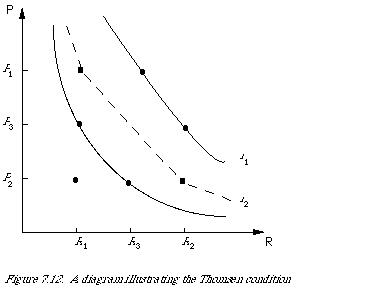Definition 3 (Thomsen condition). For every R1, R2 , R3 [[propersubset]] R and P1, P2, P3 [[propersubset]] P, (R1, P3) ~ (R3, P 2) and (R3, P1) ~ (R2, P 3) imply that (R1, P1) ~ (R2, P 2).

Intuitively this can b e reasoned as follows. The intervals R1 R3 and P2 P 3 are equivalent since an increase in the R-factor by R1 R3 and an increase in the P-factor by P2 P3 starting from (R1 , P3) lead to the same effectiveness (points on l2). It therefore follows that a decrease in each factor starting from equal effectiveness, in this case the two points (R3, R1) and (R2 , P3) on l1, should lead to equal effectiveness.

The fourth condition is one concerned with the continuity of each component. It makes precise what intuitively we would expect when considering the existence of intermediate values.

Definition 4 (Restricted Solvability). A relation >= on R x P satisfies restricted solvability provided that:

(1) whenever R, `R, R [[propersubset]] R and P, P' [[propersubset]] P for which (`R, P') >= (R, P) >= (R, P') then there exists R [[propersubset]] R s.t. (R, P') ~ (R, P);

(2) a similar condition holds on the second component.

In other words we are ensuring that the equation (R', P') ~ (R, P) is soluble for R' provided that there exist `R, R such that (`R, P') >= (R, P') >= (R, P'). An assumption of continuity of the precision and recall factors would ensure this.

The fifth condition is not limiting in any way but needs to be stated. It requires, in a precise way, that each component is essential.

Definition 5. Component R is essential if and only if there exist R1, R2 [[propersubset]] R and P1 [[propersubset]] P such that it is not the case that (R1, P1) ~ (R2, P1). A similar definition holds for P.

Thus we require that variation in one while leaving the other constant gives a variation in effectiveness.

Finally we need a technical condition which will not be explained here, that is the Archimedean property for each component. It merely ensures that the intervals on a component are comparable. For details the reader is referred to Krantz et al.

We now have six conditions on the relational structure <R x P, >= > which in the theory of measurement are necessary and sufficient conditions* for it to be an additive conjoint structure. This is enough for us to state the main representation theorem. It is a theorem asserting that if a given relational structure satisfies certain conditions (axioms), then a homomorphism into the real numbers is often referred to as a scale. Measurement may therefore be regarded as the construction of homomorphisms for empirical relational structures of interest into numerical relational structures that are useful.

In our case we can therefore expect to find real-valued functions [[Phi]]1 on R and [[Phi]]2 on P and a function F from Re x Re into Re, 1:1 in each variable, such that, for all R, R' [[propersubset]] R and P, P' [[propersubset]] P we have:

(R, P) >= (R', P') <=> F [[[Phi]]1 (R ), [[Phi]]2 (P )] >= F [[[Phi]]1 (R' ), [[Phi]]2 (P' )]

(Note that although the same symbol >= is used, the first is a binary relation on R x P, the second is the usual one on Re, the set of reals.)

In other words there are numerical scales [[Phi]]i on the two components and a rule F for combining them such that the resultant measure preserves the qualitative ordering of effectiveness. When such a representation exists we say that the structure is decomposable. In this representation the components (R and P) contribute to the effectiveness measure independently. It is not true that all relational structures are decomposable. What is true, however, is that non-decomposable structures are extremely difficult to analyse.

A further simplification of the measurement function may be achieved by requiring a special kind of non-interaction of the components which has become known as additive independence. This requires that the equation for decomposable structures is reduced to:

(R, P) >= (R', P' ) <=> [[Phi]]1 (R ) + [[Phi]]2 (P ) >= [[Phi]]1 (R' ) + [[Phi]]2 (P' )

where F is simply the addition function. An example of a non-decomposable structure is given by:

(R, P) >= (R', P') <=> [[Phi]]1 (R ) + [[Phi]]2 (P ) + [[Phi]]1 (R ) [[Phi]]2 (P ) >= [[Phi]]1 (R' ) + [[Phi]]2 (P' ) +

+ [[Phi]]1 (R' )[[Phi]]2 (P' )

* It can be shown that (starting at the other end) given an additively independent representation the properties defined in 1 and 3, and the Archimedean property are necessary. The structural conditions 4 and 5 are sufficient.

Here the term [[Phi]]1 [[Phi]]2 is referred to as the interaction term, its absence accounts for the non-interaction in the previous condition.

We are now in a position to state the main representation theorem.

Theorem

Suppose <R x P, >= > is an additive conjoint structure, then there exist functions, [[Phi]]1 from R, and [[Phi]]2 from P into the real numbers such that, for all R, R' [[propersubset]] R and P, P' [[propersubset]] P:

(R, P) >= (R', P' ) <=> [[Phi]]1 (R ) + [[Phi]]2 (P ) >= [[Phi]]1 (R' ) + [[Phi]]2 (P' )

If [[Phi]]i['] are two other functions with the same property, then there exist constants [[Theta]] > 0, [[gamma]]1, and [[gamma]]2 such that

[[Phi]]1['] = [[Theta]][[Phi]]1 + [[gamma]]1 [[Phi]]2['] = [[Theta]][[Phi]]2 + [[gamma]]2

The proof of this theorem may be found in Krantz et al..

Let us stop and take stock of this situation. So far we have discussed the properties of an additive conjoint structure and justified its use for the measurement of effectiveness based on precision and recall. We have also shown that an additively independent representation (unique up to a linear transformation) exists for this kind of relational structure. The explicit form of [[Phi]]i has been left unspecified. To determine the form of [[Phi]]i we need to introduce some extrinsic considerations. Although the representation F = [[Phi]]1 + [[Phi]]2 , this is not the most convenient form for expressing the further conditions we require of F, nor for its interpretation. So, in spite of the fact that we are seeking an additively independent representation we consider conditions on a general F. It will turn out that the F which is appropriate can be simply transformed into an additive representation. The transformation is f (F) = - (F - 1)[-1] which is strictly monotonically increasing in the range 0 <= F <= 1, which is the range of interest. In any case, when measuring retrieval effectiveness any strictly monotone transformation of the measure will do just as well.

Explicit measures of effectiveness

I shall now argue for a specific form of [[Phi]]i and F, based on a model for the user. In other words, the form [[Phi]]i and F are partly determined by the user. We start by showing how the ordering on R x P in fact induces an ordering of intervals on each factor. From Figure 7.13 we have that (R3, P1) >= (R1, P2), (R3, P1) >= (R1, P1) and (R1, P2) >= (R1, P1). Therefore the increment (interval) R1R3 is preferred to the increment P1P2. But (R2, P2) >= (R4, P1), which gives P1 P2 is preferred to R2 R4. Hence R1 R3 >=1 R2, R4 where >=1 is the induced order relation on R. We now have a method of comparing each interval on R with a fixed interval on P.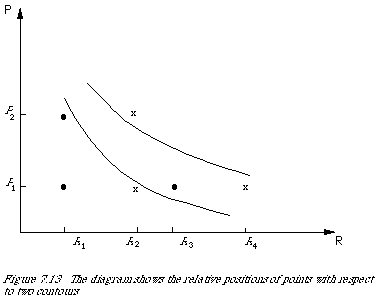Since we have assumed that effectiveness is determined by precision and recall we have committed ourselves to the importance of proportions of documents rather than absolute numbers. Consistent with this is the assumption of decreasing marginal effectiveness. Let me illustrate this with an example. Suppose the user is willing to sacrifice one unit of precision for an increase of one unit of recall, but will not sacrifice another unit of precision for a further unit increase in recall, i.e.

(R + 1, P - 1) > (R, P)

but

(R + 1, P) > (R + 2, P - 1)

We conclude that the interval between R + 1 and R exceeds the interval between P and P - 1 whereas the interval between R + 1 and R + 2 is smaller. Hence the marginal effectiveness of recall is decreasing. (A similar argument can be given for precision.) The implication of this for the shape of the curves of equal effectiveness is that they are convex towards the origin.

Finally, we incorporate into our measurement procedure the fact that users may attach different relative importance to precision and recall. What we want is therefore a parameter (ß) to characterise the measurement function in such a way that we can say: it measures the effectiveness of retrieval with respect to a user who attaches ß times as much importance to recall as precision. The simplest way I know of quantifying this is to specify the P/R ratio at which the user is willing to trade an increment in precision for an equal loss in recall.

Definition 6. The relative importance a user attaches to precision and recall is the P/R ratio at which [[partialdiff]]E/ [[partialdiff]]R = [[partialdiff]]E/ [[partialdiff]]P, where E = E(P, R) is the measure of effectiveness based on precision and recall.

Can we find a function satisfying all these conditions? If so, can we also interpret it in an intuitively simple way? The answer to both these questions is yes. It involves: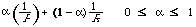The scale functions are therefore, [[Phi]]1(P) = [[alpha]](1/P), and [[Phi]]2(R) = (1 - [[alpha]]) (1/R). The 'combination' function F is now chosen to satisfy definition 6 without violating the additive independence. We get:We now have the effectiveness measure. In terms of P and R it will be: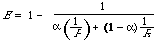To facilitate interpretation of the function, we transform according to [[alpha]] = 1/(ß + 1), and find that [[partialdiff]]E/ [[partialdiff]]R = [[partialdiff]]E/ [[partialdiff]]P when P/R = ß. If A is the set of relevant documents and B the set of retrieval documents, then: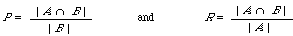E now gives rise to the following special cases:

(1) When [[alpha]] = 1/2 (ß = 1) E = |A [[Delta]] B | / (|A | + |B |), a normalised symmetric difference between sets A and B (A [[Delta]] B = A [[union]] B - A [[intersection]] B). It corresponds to a user who attaches equal importance to precision and recall.

(2) E -> 1 - R when [[alpha]] -> 0 (ß -> *), which corresponds to a user who attaches no important to precision.

(3) E -> 1 - P when [[alpha]] -> 1 (ß -> 0), which corresponds to a user who attaches no importance to recall.

It is now a simple matter to show that certain other measures given in the literature are special cases of the general form E. By the representation theorem, the [[Phi]]i 's are uniquely determined up to a linear transformation, that is, [[Phi]]i['] = [[Theta]][[Phi]]i + [[gamma]]i would serve equally well as scale functions. If we now set [[Phi]]1['] = 2[[Phi]]1 - 1/2, [[Phi]]2['] = 2[[Phi]]2 - 1/2, and ß = 1 then we have: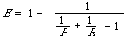which is the measure recommended by Heine.

One final example is the measure suggested by Vickery in 1965 which was documented by Cleverdon et al.. Here we set:which is Vickery's measure (apart from a scale factor of 100).

To summarise, we have shown that it is reasonable to assume that effectiveness in terms of precision and recall determines an additive conjoint structure. This guarantees the existence of an additively independent representation. We then found the representation satisfying some user requirements and also having special cases which are simple to interpret.

The analysis is not limited to the two factors precision and recall, it could equally well be carried out for say the pair fallout and recall. Furthermore, it is not necessary to restrict the model to two factors. If appropriate variables need to be incorporated the model readily extends to n factors. In fact, for more than two dimensions the Thomsen condition is not required for the representation theorem.

Presentation of experimental results

In my discussion of micro-, macro-evaluation, and expected search length, various ways of averaging the effectiveness measure of the set of queries arose in a natural way. I now want to examine the ways in which we can summarise our retrieval results when we have no a priori reason to suspect that taking means is legitimate.

In this section the discussion will be restricted to single number measures such as a normalised symmetric difference, normalised recall, etc. Let us use Z to denote any arbitrary measure. The test queries will be Qi and n in number. Our aim in all this is to make statements about the relative merits of retrieval under different conditions a,b,c, . . . in terms of the measure of effectiveness Z. The 'conditions' a,b,c, . . . may be different search strategies, or information structures, etc. In other words, we have the usual experimental set-up where we control a variable and measure how its change influences retrieval effectiveness. For the moment we restrict these comparisons to one set of queries and the same document collection.

The measurements we have therefore are {Za(Q1), Za(Q2), . . . }, {Zb(Q1), Zb(Q2), . . . }, {Zc(Q1), Zc(Q2), . . . }, . . . where Zx(Q1) is the value of Z when measuring the effectiveness of the response to Qi under conditions x. If we now wish to make an overall comparison between these sets of measurements we could take means and compare these. Unfortunately, the distributions of Z encountered are far from bell-shaped, or symmetric for that matter, so that the mean is not a particularly good 'average' indicator. The problem of summarising IR data has been a hurdle every since the beginning of the subject. Because of the non-parametric nature of the data it is better not to quote a single statistic but instead to show the variation in effectiveness by plotting graphs. Should it be necessary to quote 'average' results it is important that they are quoted alongside the distribution from which they are derived.

There are a number of ways of representing sets of Z-values graphically. Probably the most obvious one is to use a scatter diagram, where the x-axis is scaled for Za and the y-axis for Zb and each plotted point is the pair (Za(Qi), Zb(Qi)). The number of points plotted will equal the number of queries. If we now draw a line at 45[[ring]] to the x-axis from the origin we will be able to see what proportion of the queries did better under condition a than under condition b. There are two disadvantages to this method of representation: the comparison is limited to two conditions, and it is difficult to get an idea of the extent to which two conditions differ.

A more convenient way of showing retrieval results of this kind is to plot them as cumulative frequency distributions, or as they are frequently called by statisticians empirical distribution functions. Let {Z(Q1), Z(Q2), . . . , Z(Qn)} be a set of retrieval results then the empirical distribution function F(z) is a function of z which equals the proportion of Z(Qi)'s which are less than or equal to z. To plot this function we divide the range of z into intervals. If we assume that 0 <= z <= 1, then a convenient set of intervals is ten. The distributions will take the general shape as shown in Figure 7.14. When the measure Z is such that the smaller its value the more effective the retrieval, then the higher the curve the better. It is quite simple to read off the various quantiles. For example, to find the median we only need to find the z-value corresponding to 0.5 on the F(z) axis. In our diagrams they are 0.2 and 0.4 respectively for conditions a and b.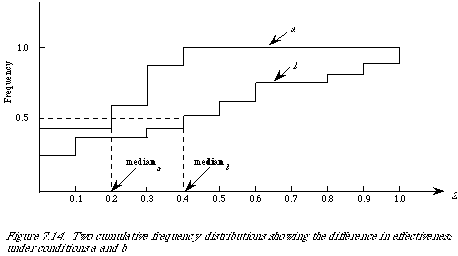I have emphasised the measurement of effectiveness from the point of view of the user. If we now wish to compare retrieval on different document collections with different sets of queries then we can still use these measures to indicate which system satisfies the user more. On the other hand, we cannot thereby establish which system is more effective in its retrieval operations. It may be that in system A the sets of relevant documents constitute a smaller proportion of the total set of documents than is the case in system B. In other words, it is much harder to find the relevant documents in system B than in system A. So, any direct comparison must be weighted by the generality measure which gives the number of relevant documents as a proportion of the total number of documents. Alternatively one could use fallout which measures the proportion of non-relevant documents retrieved. The important point here is to be clear about whether we are measuring user satisfaction or system effectiveness.

Significance tests

Once we have our retrieval effectiveness figures we may wish to establish that the difference in effectiveness under two conditions is statistically significant. It is precisely for this purpose that many statistical tests have been designed. Unfortunately, I have to agree with the findings of the Comparative Systems Laboratory in 1968, that there are no known statistical tests applicable to IR. This may sound like a counsel of defeat but let me hasten to add that it is possible to select a test which violates only a few of the assumptions it makes. Two good sources which spell out the pre-conditions for non-parametric tests are Siegal and Conover. A much harder but also more rewarding book on non-parametrics is Lehmann.

Parametric tests are inappropriate because we do not know the form of the underlying distribution. In this class we must include the popular t-test. The assumptions underlying its use are given in some detail by Siegel (page 19), needless to say most of these are not met by IR data. One obvious failure is that the observations are not drawn from normally distributed populations.

On the face of it non-parametric tests might provide the answer. There are some tests for dealing with the case of related samples. In our experimental set-up we have one set of queries which is used in different retrieval environments. Therefore, without questioning whether we have random samples, it is clear that the sample under condition a is related to the sample under condition b. When in this situation a common test to use has been the Wilcoxon Matched-Pairs test. Unfortunately again some important assumptions are not met. The test is done on the difference Di = Za (Qi) - Zb (Qi), but it is assumed that Di is continuous and that it is derived from a symmetric distribution, neither of which is normally met in IR data.

It seems therefore that some of the more sophisticated statistical tests are inappropriate. There is, however, one simple test which makes very few assumptions and which can be used providing its limitations are noted. This one is known in the literature as the sign test (Siegel, page 68 and Conover, page 121). It is applicable in the case of related samples. It makes no assumptions about the form of the underlying distribution. It does, however, assume that the data are derived from a continuous variable and that the Z (Qi) are statistically independent. These two conditions are unlikely to be met in a retrieval experiment. Nevertheless, given that some of the conditions are not met, it can be used conservatively.

The way it works is as follows: Let {Za (Q1), Za (Q2), . . .,}, {Zb (Q1), Zb (Q2). . .,} be our two sets of measurements under conditions a and b respectively. Within each pair (Za (Qi), Zb (Qi)) a comparison is made, and each pair is classified as ' + ' if Za (Qi) > Zb (Qi), as ' - ' if Za (Qi) < Zb (Qi) or 'tie' if Za (Qi) = Za (Qi). Pairs which are classified as 'tie' are removed from the analysis thereby reducing the effective number of measurements. The null hypothesis we wish to test is that:

P (Za > Zb ) = P (Za < Zb ) = /2

Under this hypothesis we expect the number of pairs which have Za > Zb to equal the number of pairs which have Za < Zb . Another way of stating this is that the two populations from which Za and Zb are derived have the same median.

In IR this test is usually used as a one-tailed test, that is, the alternative hypothesis prescribes the superiority of retrieval under condition a over condition b, or vice versa. A table for small samples n <= 25 giving the probability under the null hypothesis for each possible combination of '+''s and '-''s may be found in Siegal (page 250). To give the reader a feel for the values involved: in a sample of 25 queries the null hypothesis will be rejected at the 5 per cent level if there are at least 14 differences in the direction predicted by the alternative hypothesis.

The use of the sign test raises a number of interesting points. The first of these is that unlike the Wilcoxon test it only assumes that the Z's are measured on an ordinal scale, that is, the magnitude of |Za - Zb | is not significant. This is a suitable feature since we are usually only seeking to find which strategy is better in an average sense and do not wish the result to be unduly influenced by excellent retrieval performance on one query. The second point is that some care needs to be taken when comparing Za and Zb. Because our measure of effectiveness can be calculated to infinite precision we may be insisting on a difference when in fact it only occurs in the tenth decimal place. It is therefore important to decide beforehand at what value of [[propersubset]] we will equate Za and Zb when |Za - Zb | <= [[propersubset]].

Finally, although I have just explained the use of the sign test in terms of single number measures, it is also used to detect a significant difference between precision-recall graphs. We now interpret the Z's as precision values at a set of standard recall values. Let this set be SR = {0,1, 0.2, . . ., 1.0}, then corresponding to each R[[propersubset]] SR we have a pair (Pa (R) Pb (R)). The Pa's and Pb's are now treated in the same way as the Za's and Zb's. Note that when doing the evaluation this way, the precision-recall values will have already been averaged over the set of queries by one of the ways explained before.

Bibliographic remarks

Quite a number of references to the work on evaluation have already been given in the main body of the chapter. Nevertheless, there are still a few important ones worth mentioning.

Buried in the report by Keen Digger (Chapter 16) is an excellent discussion of the desirable properties of any measure of effectiveness. It also gives a checklist indicating which measure satisfies what. It is probably worth repeating here that Part I of Robertson's paper contains a discussion of measures of effectiveness based on the 'contingency' table as well as a list showing who used what measure in their experiments. King and Bryant have written a book on the evaluation of information services and products emphasising the commercial aspects. Goffman and Newill describe a methodology for evaluation in general.

A parameter which I have mentioned in passing but which deserves closer study in generality. Salton has recently done a study of its effect on precision and fallout for different sized document collections.

The trade-off between precision and recall has for a long time been the subject of debate. Cleverdon who has always been involved in this debate has now restated his position. Heine, in response to this, has attempted to further clarify the trade-off in terms of the Swets model.

Guazzo and Cawkell describe an approach to the measurement of retrieval effectiveness based on information theory.

The notion of relevance has at all times attracted much discussion. An interesting early philosophical paper on the subject is by Weiler. Goffman has done an investigation of relevance in terms of Measure Theory. And more recently Negoita has examined the notion in terms of different kinds of logics.

A short paper by Good which is in sympathy with the approach based on a theory of measurement given here, discusses the evaluation of retrieval systems in terms of expected utility.

One conspicuous omission from this chapter is any discussion of cost-effectiveness. The main reason for this is that so far very little of importance can be said about it. A couple of attempts to work out mathematical cost models for IR are Cooper and Marschak.

References

1. COOPER, W.S., 'On selecting a measure of retrieval effectiveness', Part 1: 'The "subjective" philosophy of evaluation', Part 2: 'Implementation of the philosophy', Journal of the American Society for Information Science, 24, 87-100 and 413-424 (1973).

2. JARDINE, N. and van RIJSBERGEN, C.J., 'The use of hierarchic clustering in information retrieval', Information Storage and Retrieval, 7, 217-240 (1971).

3. HEINE, M.H., 'Distance between sets as an objective measure of retrieval effectiveness', Information Storage and Retrieval, 9, 181-198 (1973).

4. COOPER, W.S., 'A definition of relevance for information retrieval', Information Storage and Retrieval, 7, 19-37 (1971).

5. BELNAP, N.D., An analysis of questions: Preliminary report, Scientific Report TM-1287, SDC, Santa Monica, California (1963).

6. BELNAP, N.D. and STEEL, T.B., The Logic of Questions and Answers, Yale University Press, New Haven and London (1976).

7. CHANG, C.L. and LEE, R.C.T., Symbolic Logic and Mechanical Theorem Proving, Academic Press, New York (1973).

8. SARACEVIC, T., 'Relevance: A review of and a framework for the thinking on the notion information science', Journal of the American Society for Information Science,

26, 321-343 (1975).

9. ROBERTSON, S.E., 'The probabilistic character of relevance', Information Processing and Management, 13, 247-251 (1977).

10. van RIJSBERGEN, C.J., 'Retrieval effectiveness', In: Progress in Communication Sciences, Vol. 1 (Edited by Melvin J. Voigt), 91-118 (1979).

11. KEEN, E.M., 'Evaluation parameters'. In Report ISR-13 to the National Science Foundation, Section IIK, Cornell University, Department of Computer Science (1967).

12. SWETS, J.A., 'Information retrieval systems', Science, 141, 245-250 (1963).

13. SWETS, J.A., Effectiveness of Information Retrieval Methods, Bolt, Beranek and Newman, Cambridge, Massachusetts (1967).

14. BROOKES, B.C., 'The measure of information retrieval effectiveness proposed by Swets', Journal of Documentation, 24, 41-54 (1968).

15. ROBERTSON, S.E., 'The parametric description of retrieval tests, Part 2: 'Overall measures', Journal of Documentation, 25, 93-107 (1969).

16. BOOKSTEIN, A., 'When the most "pertinent" document should not be retrieved - an analysis of the Swets Model', Information Processing and Management, 13, 377-383 (1977).

17. ROBERTSON, S.E. and TEATHER, D., 'A statistical analysis of retrieval tests: a Bayesian approach', Journal of Documentation, 30, 273-282 (1974).

18. EFRON, B. and MORRIS, C., 'Stein's parador in statistics', Scientific American, 236, 119-127 (1977).

19. ROBERTSON, S.E., 'A Theoretical Model of the Retrieval Characteristics of Information Retrieval Systems', Ph.D. Thesis, University College London (1975).

20. COOPER, W.S., 'Expected search length: A single measure of retrieval effectiveness based on weak ordering action of retrieval systems', Journal of the American Society for Information Science, 19, 30-41 (1968).

21. SENKO, M.E., Information storage and retrieval systems, In: Advances in Information Systems Science, (Edited by J. Tou) Plenum Press, New York (1969).

22. SOERGEL, D., 'Is user satisfaction a hobgoblin?', Journal of the American Society for Information Science, 27, 256-259 (1976).

23. SALTON, G., Automatic Information Organization and Retrieval, McGraw-Hill, New York (1968).

24. KRANTZ, D.H., LUCE, R.D., SUPPES, P. and TVERSKY, A., Foundations of Measurement, Volume 1, Additive and Polynomial Representation, Academic Press, London and New York (1971).

25. ELLIS, B., Basic Concepts of Measurement, Cambridge University Press, London (1966).

26. LIEBERMAN, B., Contemporary Problems in Statistics, Oxford University Press, New York (1971).

27. CLEVERDON, C.W., MILLS, J. and KEEN, M., Factors Determining the Performance of Indexing Systems, Volume I - Design, Volume II - Test Results, ASLIB Cranfield Project, Cranfield (1966).

28. Comparative Systems Laboratory, An Inquiry into Testing of Information Retrieval Systems, 3 Volumes, Case-Western Reserve University (1968).

29. SIEGEL, S., Nonparametric Statistics for the Behavioural Sciences, McGraw-Hill, New York (1956).

30. CONOVER, W.J., Practical Nonparametric Statistics, Wiley, New York (1971).

31. LEHMANN, E.L., Nonparametrics: Statistical Methods Based on Ranks, Holden-Day Inc., San Francisco, California (1975).

32. KEEN, E.M. and DIGGER, J.A., Report of an Information Science Index Languages Test, Aberystwyth College of Librarianship, Wales (1972).

33. ROBERTSON, S.E., 'The parametric description of retrieval tests', Part I: 'The basic parameters', Journal of Documentation, 25, 1-27 (1969).

34. KING, D.W. and BRYANT, E.C., The Evaluation of Information Services and Products, Information Resources Press, Washington (1971).

35. GOFFMAN, W. and NEWILL, V.A., 'A methodology for test and evaluation of information retrieval systems', Information Storage and Retrieval, 3, 19-25 (1966).

36. SALTON, G., 'The "generality" effect and the retrieval evaluation for large collections', Journal of the American Society for Information Science, 23, 11-22 (1972).

37. CLEVERDON, C.W., 'On the inverse relationship of recall and precision', Journal of Documentation, 28, 195-201 (1972).

38. HEINE, M.H., 'The inverse relationship of precision and recall in terms of the Swets' model', Journal of Documentation, 29, 81-84 (1973).

39. GUAZZO, M., 'Retrieval performance and information theory', Information Processing and Management, 13, 155-165 (1977).

40. CAWKELL, A.E., 'A measure of "Efficiency Factor" - communication theory applied to document selection systems', Information Processing and Management, 11, 243-248 (1975).

41. WEILER, G., 'On relevance', Mind, LXXI, 487-493 (1962).

42. GOFFMAN, W., 'On relevance as a measure', Information Storage and Retrieval, 2, 201-203 (1964).

43. NEGOITA, C.V., 'On the notion of relevance', Kybernetes, 2, 161-165 (1973).

44. GOOD, I.J., 'The decision-theory approach to the evaluation of information retrieval systems', Information Storage and Retrieval, 3, 31-34 (1967).

45. COOPER, M.D., 'A cost model for evaluating information retrieval systems', Journal of the American Society for Information Science, 23, 306-312 (1972).

46. MARSCHAK, J., 'Economics of information systems', Journal of the American Statistical Association, 66, 192-219 (1971).